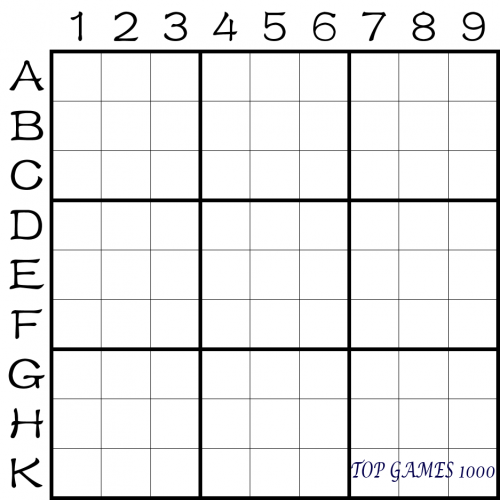# The Origin of Sudoku and Basic Knowledge of Sudoku Rules

1-1 The Origin of Sudoku

Sudoku is said to be a creation of Euler, but its exact origin is unknown. The author tends to believe that it is the result of the fusion and evolution of various intellectual and mathematical games over a long period of time.

1-2 The Rules of Sudoku

Basic rules: Fill in the blanks with numbers from 1 to 9 so that each row, column, and block contains no duplicate numbers. Only one number can be filled in each grid.

This version of the rules is the most basic and is also known as the standard Sudoku rules. Some modified versions of Sudoku may add additional rules on top of the standard Sudoku rules.

Note 1: Non-nine-order Sudoku without rule changes is also considered standard Sudoku.

Note 2: There is currently some controversy over whether Compound Sudoku is a modified version of Sudoku.

1-3 Sudoku Terminology

There are several elements related to Sudoku, including:

1. Row:

Horizontal rows are labeled A to I from top to bottom. In some materials, letters A to H and K may be used instead of I due to its similarity to number 1. Alternatively, rows may be labeled with “R” (Row) followed by the row number, such as R5 for the fifth row.

2. Column:

Vertical columns are labeled 1 to 9 from left to right. In some materials, “C” (Column) may be used instead of numbers to represent columns.3. Block:

Each thick-lined section is called a block and is represented by a Chinese character. The nine blocks are named as shown in the figure below. The block marked with the number 3 is the third block, and so on.

READ  The Longest and Largest Sudoku Solution

4. Row Unit/Column Unit

Three rows adjacent to each other and able to form three blocks are called a row unit, such as the ABC rows. Similarly, three columns adjacent to each other and able to form three blocks are called a column unit, such as the 789 columns.

5. Coordinate:

The combination of the row and column labels represents the coordinate of a grid. For example, the coordinate of the number 4 in the figure is E2. If the RC method is used, it would be R5C2.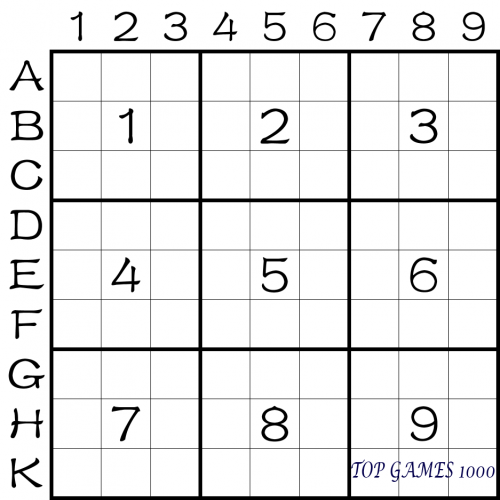6. Conjugate:

Conjugates refer to grids that have a relationship with a specified grid, in that they cannot have the same number according to the rules. In the figure below, the gray grid is conjugate to B2, so none of them can be number 1.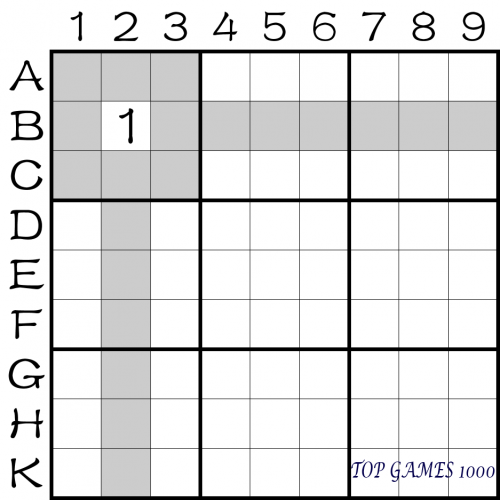7. Initial board, final board, solution, known numbers:

The initial board refers to the starting state of the puzzle. The final board refers to the completed state of the puzzle, as shown in the figure below. Broadly speaking, an initial board may have multiple final boards, but such puzzles are considered unqualified. Usually, Sudoku puzzles are designed with a unique solution within the specified rules, which is called the solution of the puzzle. When creating a Sudoku puzzle, the most basic requirement, in addition to complying with the rules, is to have a “unique solution”.

Known numbers broadly refer to all the numbers that have been determined, including those given in the initial board and those that have been filled in. Strictly speaking, only the numbers given in the initial board are considered known numbers. In the figure below, there are 28 known numbers. The number and position of the known numbers are usually irrelevant to the difficulty level of the puzzle.

Note: To make a puzzle qualified, it must have at least 17 known numbers.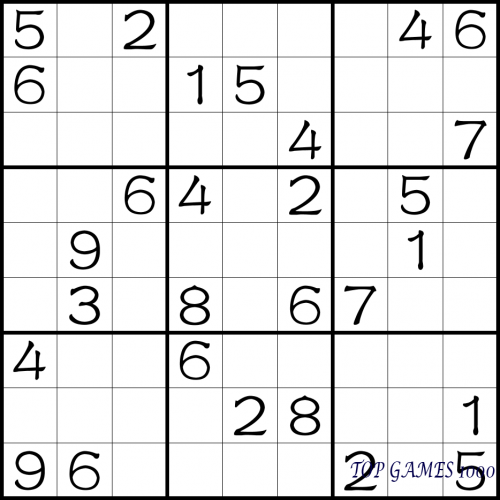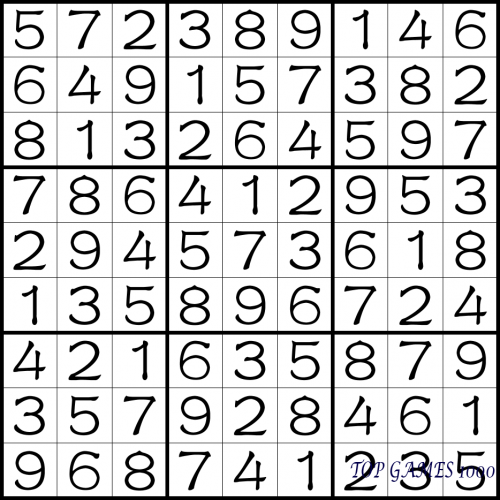8. Blocking point, difficulty:

Sudoku solving techniques can be divided into levels such as basic, advanced, high-level, theoretical, etc. Basic techniques can solve more than 99% of the steps, while the steps that cannot be solved by basic techniques are called blocking points.

A puzzle may have multiple blocking points or a single blocking point that requires multiple steps to solve. The difficulty level of a puzzle is determined by the highest technique required to solve it, in addition to observation difficulty, which means that puzzles using the same techniques may have different levels of difficulty due to different perspectives.